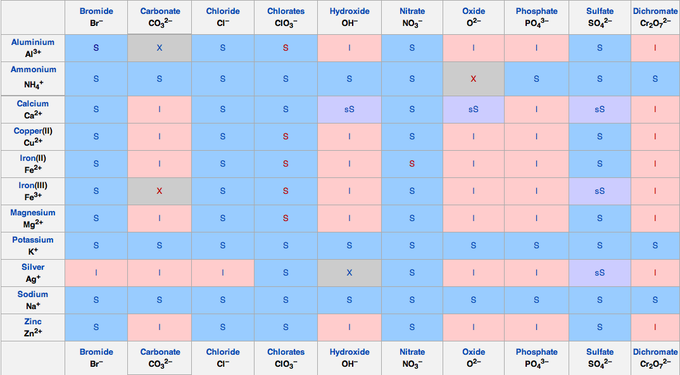## Precipitation Reactions

#### Learning Objective

• Distinguish ways to write precipitation reactions (complete ionic equation and net ionic equation) and use a solubility table to determine whether a precipitation reaction will occur

#### Key Points

• A precipitation reaction refers to the formation of an insoluble salt when two solutions containing soluble salts are combined. The insoluble salt that falls out of solution is known as the precipitate, hence the reaction’s name.
• Precipitation reactions can help determine the presence of various ions in solution.
• A solubility table can be used to predict precipitation reactions.

#### Terms

• precipitationthe process of an insoluble salt forming from its aqueous ions and falling out of solution
• net ionic equationa method or writing a precipitation reaction without spectator ions

Precipitation refers to a chemical reaction that occurs in aqueous solution when two ions bond together to form an insoluble salt, which is known as the precipitate.

A precipitation reaction can occur when two solutions containing different salts are mixed, and a cation/anion pair in the resulting combined solution forms an insoluble salt; this salt then precipitates out of solution.

The following is a common laboratory example of a precipitation reaction. Aqueous silver nitrate (AgNO3) is added to a solution containing potassium chloride (KCl), and the precipitation of a white solid, silver chloride (AgCl), is observed:

AgNO3 (aq) + KCl (aq) → AgCl (s) + KNO3(aq)

Note that the product silver chloride is the precipitate, and it is designated as a solid. This reaction can be also be written in terms of the individual dissociated ions in the combined solution. This is known as the complete ionic equation:

Ag+ (aq) + NO3(aq) + K+ (aq) + Cl(aq) → AgCl (s) + K+ (aq) + NO3(aq)

A final way to represent a precipitation reaction is known as the net ionic equation. In this case, any spectator ions (those that do not contribute to the precipitation reaction) are left out of the formula completely. Without the spectator ions, the reaction equation simplifies to the following:

Ag+(aq) + Cl(aq) → AgCl (s)

Observing precipitation reactions can be useful in the laboratory to determine the presence of various ions in solution. For instance, if silver nitrate is added to a solution of an unknown salt and a precipitate is observed, the unknown solution might contain chloride (Cl).

Lastly, to make predictions about precipitation reactions, it is important to remember solubility rules. The following solubility chart gives a useful summary:Solubility chartTo determine the solubility of an given salt, find the cationic component along the left-hand side, match it to the anionic component along the top, then check to see if it is S – soluble, I – insoluble, or sS – slightly soluble.